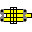#Addition and subtraction

Usually it is not practical to perform single addition or subtraction with slide rule. It can make some sense to do it in chain repeated calculations.

Addition (and subtraction) is based on a formulas

(u+v)=v*(u/v+1)
(u-v)=v*(u/v-1)

## u+v, u-v (C, D)

Let v be operand with smaller mantissa.
1. Place left index of C scale over v on D scale.
2. Read the number (u/v -- be careful with digit count) from scale C over u on scale D.
3. Add 1 to it (u/v+1) and move cursor there.
4. Read u+v from D scale under cursor.
If (u/v+1) goes out of scale try to place right index of C over u on d scale.

Subtraction is done essentially the same way, but instead of adding 1 you should (surprise!) subtract it.

### Example

Calculate 2.3+3.5.
• Place left index of C scale ofer 2.3 (v).
• Read 1.52 (u/v) from C over 3.5.
• Add 1 to 1.52 getting 2.52.
• Move cursor to 2.52 on C scale.
• Read 5.8 from D scale under cursor.

## u+v, u-v (L)

If slide rule posesses L scale on the slide then this this straightforward method will work fine.
• Move left index of L scale to left index of D scale (or any other scale on the slide)
• Move hairline to u on L scale
• Move index of L under hairline
• Move hairline to v on L scale
• Move left index of L scale to left index of D scale (or any other scale on the slide)
• Read the result u+v from L under the hairline
This algorithm requires 3 slide movements but the fact that obly ONE scale is used makes it worth mentioning.

## u2+v2 (C, D, A, B)

Method is based on equality u2+v2=v2*(u2/v2+1). Again, let v be operand with smaller mantissa.
1. Place left index of C scale over v on D scale.
2. Read the number (u2/v2 -- be careful with digit count) from scale B over u on scale D.
3. Add 1 to it (u2/v2+1) and move cursor to this number on scale B.
4. Read u2+v2 from A scale under cursor.
or/and
5. Read √(u2+v2) from D scale under cursor.
This algorithm can be used to calculate module of complex number. Or hypothenuse length. Or whatever involving sum of two squares. See also
trigonometry page for different method of finding hypothenuse.

## 1/(1/u+1/v)

Method is based on equality 1/(1/u+1/v)=1/(u(u/v+1)).
1. Move 1C to u on D.
2. Move cursor to v on D.
3. Read x:=u/v from CI under cursor.
4. Add 1 to x and move cursor to 1+x on D.
5. Read 1/u+1/v from C under cursor
or
Read 1/(1/u+1/v) from CI under cursor.

[ Index page | Emulator | Notation | Deframe | Feedback ]